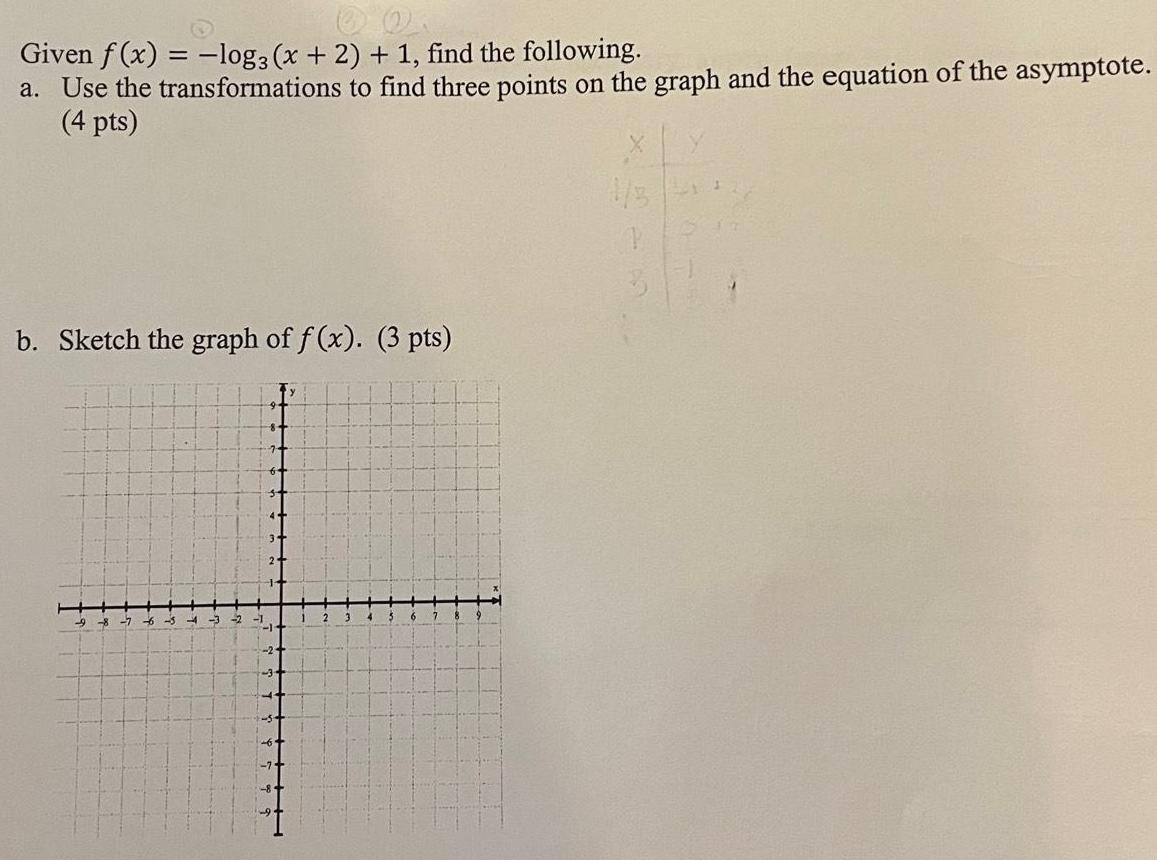Question:

# Given f(x) = -log3 (x + 2) + 1, find the following. a. Use

Last updated: 8/14/2022Given f(x) = -log3 (x + 2) + 1, find the following. a. Use the transformations to find three points on the graph and the equation of the asymptote. b. Sketch the graph of f(x).